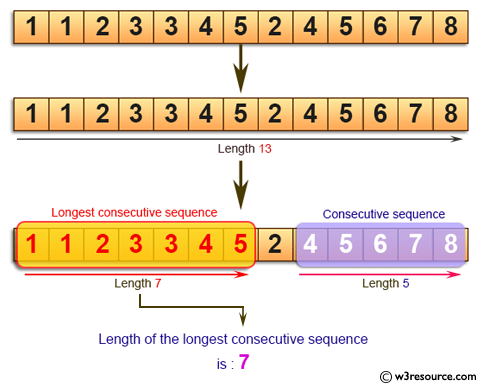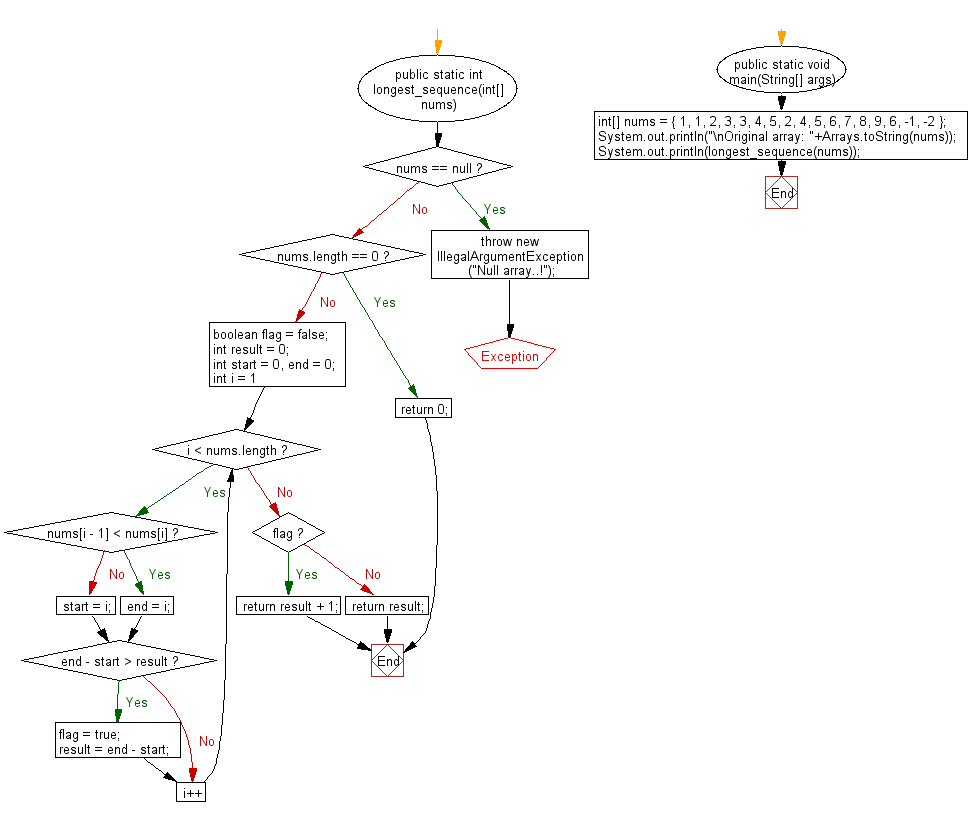﻿ Java programming exercises: Find the length of the longest consecutive sequence of a given array of integers - w3resource# Java Exercises: Find the length of the longest consecutive sequence of a given array of integers

## Java Basic: Exercise-170 with Solution

Write a Java program to find the length of the longest consecutive sequence of a given array of integers.

Pictorial Presentation:Sample Solution:

Java Code:

``````import java.util.*;
public class Solution {
public static int longest_sequence(int[] nums) {
if (nums == null) {
throw new IllegalArgumentException("Null array..!");
}
if (nums.length == 0) {
return 0;
}
boolean flag = false;
int result = 0;
int start = 0, end = 0;
for (int i = 1; i < nums.length; i++) {
if (nums[i - 1] < nums[i]) {
end = i;
} else {
start = i;
}
if (end - start > result) {
flag = true;
result = end - start;
}
}
if (flag)
{
return result + 1;
}
else
{
return result;
}
}

public static void main(String[] args) {
int[] nums = { 1, 1, 2, 3, 3, 4, 5, 2, 4, 5, 6, 7, 8, 9, 6, -1, -2 };
System.out.println("\nOriginal array: "+Arrays.toString(nums));
System.out.println(longest_sequence(nums));
}

}
```
```

Sample Output:

```Original array: [1, 1, 2, 3, 3, 4, 5, 2, 4, 5, 6, 7, 8, 9, 6, -1, -2]
7
```

Flowchart:Java Code Editor:

What is the difficulty level of this exercise?

﻿

## Java: Tips of the Day

Directory Content:

Java allows you to get the names of all subdirectories and files in a folder as an array, which can then be sequentially viewed.

```import java.io.*;

public class ListContents {
public static void main(String[] args) {
File file = new File("//home//user//Documents/");
String[] files = file.list();

System.out.println("Listing contents of " + file.getPath());
for(int i=0 ; i < files.length ; i++)
{
System.out.println(files[i]);
}
}
}
```